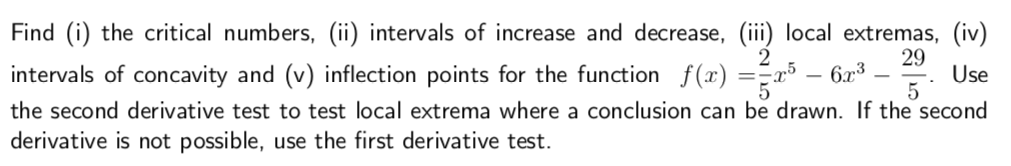# Find (i) the critical numbers, (ii) intervals of increase and decrease, (ii) local extremas, (iv) 29 intervals of concavity and (v) inflection points for the function ()Use the second derivative test to test local extrema where a conclusion can be drawn. If the second derivative is not possible, use the first derivative test.

Questionhelp_outlineImage TranscriptioncloseFind (i) the critical numbers, (ii) intervals of increase and decrease, (ii) local extremas, (iv) 29 intervals of concavity and (v) inflection points for the function ()Use the second derivative test to test local extrema where a conclusion can be drawn. If the second derivative is not possible, use the first derivative test. fullscreen

### Want to see this answer and more?

Experts are waiting 24/7 to provide step-by-step solutions in as fast as 30 minutes!*

*Response times vary by subject and question complexity. Median response time is 34 minutes and may be longer for new subjects.
Tagged in
MathCalculus

### Derivative